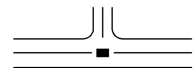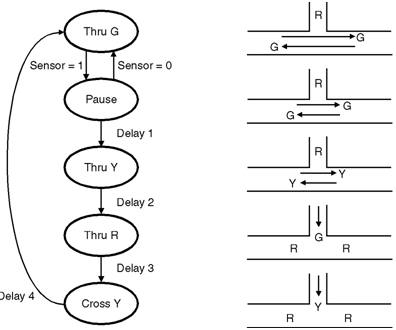Home > finite state machines > FSM Applications > Traffic Light Controller

# Traffic Light Controller

Traffic Light Controller for a junction of three roads.

Assumptions for Traffic Light Controller:

1. T intersection as shown in Figure below.2. Default to green on main road.
3. Sensor enables green for cross street.
4. Delay switching for right- turn- on- red from cross street.
5. Programmable delays.

State machine level :

1. Stay in thru G state until sensor is activated.
2. Wait in pause state to see if sensor deactivates. (right- turn- on- red).
3. Then proceed through sequence, waiting in each state for specified time delay.
4. In each state, provide appropriate control signals for lights.
5. 5 states, so at least 3 flip-flop.
Figure (b) : State diagram for three roads TLC Detailed state diagram and state table :Table P. 4.11.1 : State table Current state S2S1S0 Inputs sd1d2d3d4 Outputs TEN tGtytrxGxyxr Next state ns2 ns1 ns0 0 0 0 X X X X 0 1 0 0 0 0 1 0 0 0 0 0 0 1 X X X X 0 1 0 0 0 0 1 0 0 1 0 0 1 0 X X X X 0 1 0 0 0 0 1 0 0 0 0 0 1 1 0 X X X 1 1 0 0 0 0 1 0 0 1 0 0 1 1 1 X X X 0 1 0 0 0 0 1 0 1 0 0 1 0 X X 0 X X 1 0 1 0 0 0 1 0 1 0 0 1 0 X X 1 X X 1 0 0 1 1 0 0 0 1 1 0 1 1 X X X 0 X 0 0 0 1 1 0 0 0 1 1 0 1 1 X X X 1 X 0 0 0 1 1 0 0 1 0 0 1 0 0 X X X X 0 1 0 0 1 0 1 0 1 0 0 1 0 0 X X X X 1 0 0 0 1 0 1 0 0 0 0 VHDL code for Traffic Light Controller :```library ieee;
use ieee.std_logic_1164.all;
use ieee.std_logic_unsigned.all;
entity trafficv is
port (reset, sensor, clk: in std_logic;
d1, d2, d3, d4: in std_ logic_vector( 3 downto 0);
tg, ty, tr, xg, xy, xr: out std_logic);
end trafficv;
architecture trafficv_arch of trafficv is
type state_type is (thrug, pause, thruy, thrur, crossy);
signal state: state_type;
signal timer: std_logic_vector( 3 downto 0);
signal ten: std_logic;
begin
timer_process: process
begin
if ten = '0' then
timer <= "0000";
else
timer <= timer + "0001";
end if;
wait until clk = '1';
end process;
state_machine_process: process
begin
if reset = '1' then
state <= thrug; ten <= '0';
else
if state = thrug and sensor = '0' then
state <= thrug; ten <= '0';
elsif state = thrug and sensor = '1' then
state <= pause; ten <= '0';
elsif state = pause and sensor = '0' then
state <= thrug; ten <= '0';
elsif state = pause and sensor = '1' and timer /= d1 then
state <= pause; ten <= '1';
elsif state = pause and sensor = '1' and timer = d1 then
state <= thruy; ten <= '0';
elsif state = thruy and timer /= d2 then
state <= thruy; ten <= '1';
elsif state = thruy and timer = d2 then
state <= thrur; ten <= '0';
elsif state = thrur and timer /= d3 then
state <= thrur; ten <= '1';
elsif state = thrur and timer = d3 then
state <= crossy; ten <= '0';
elsif state = crossy and timer /= d4 then
state <= crossy; ten <= '1';
elsif state = crossy and timer = d4 then
state <= thrug; ten <= '0';
end if;
end if;
wait until clk = '1';
end process;
tg <= '1' when (state = thrug) or (state = pause) else '0';
ty <= '1' when (state = thruy) else '0';
tr <= '1' when (state = thrur) else '0';
xg <= '1' when (state = thrur) else '0';
xy <= '1' when (state = crossy) else '0';
xr <= '1' when (state = thrug) or (state = pause) or (state = thruy) else '0';
end trafficv_ arch;

```